# analytic geometry

Also found in: Dictionary, Thesaurus, Wikipedia.

## analytic geometry,

branch of geometrygeometry
[Gr.,=earth measuring], branch of mathematics concerned with the properties of and relationships between points, lines, planes, and figures and with generalizations of these concepts.
in which points are represented with respect to a coordinate system, such as Cartesian coordinatesCartesian coordinates
[for René Descartes], system for representing the relative positions of points in a plane or in space. In a plane, the point P is specified by the pair of numbers (x,y
, and in which the approach to geometric problems is primarily algebraic. Its most common application is in the representation of equations involving two or three variables as curves in two or three dimensions or surfaces in three dimensions. For example, the linear equation ax+by+c=0 represents a straight line in the xy-plane, and the linear equation ax+by+cz+d=0 represents a plane in space, where a, b, c, and d are constant numbers (coefficients). In this way a geometric problem can be translated into an algebraic problem and the methods of algebra brought to bear on its solution. Conversely, the solution of a problem in algebra, such as finding the roots of an equation or system of equations, can be estimated or sometimes given exactly by geometric means, e.g., plotting curves and surfaces and determining points of intersection.

In plane analytic geometry a line is frequently described in terms of its slope, which expresses its inclination to the coordinate axes; technically, the slope m of a straight line is the (trigonometric) tangent of the angle it makes with the x-axis. If the line is parallel to the x-axis, its slope is zero. Two or more lines with equal slopes are parallel to one another. In general, the slope of the line through the points (x1, y1) and (x2, y2) is given by m= (y2y1) / (x2x1). The conic sections are treated in analytic geometry as the curves corresponding to the general quadratic equation ax2+bxy+cy2+dx+ey+f=0, where a, b, … , f are constants and a, b, and c are not all zero.

In solid analytic geometry the orientation of a straight line is given not by one slope but by its direction cosines, λ, μ, and ν, the cosines of the angles the line makes with the x-, y-, and z-axes, respectively; these satisfy the relationship λ222= 1. In the same way that the conic sections are studied in two dimensions, the 17 quadric surfaces, e.g., the ellipsoid, paraboloid, and elliptic paraboloid, are studied in solid analytic geometry in terms of the general equation ax2+by2+cz2+dxy+exz+fyz+px+qy+rz+s=0.

The methods of analytic geometry have been generalized to four or more dimensions and have been combined with other branches of geometry. Analytic geometry was introduced by René DescartesDescartes, René
, Lat. Renatus Cartesius, 1596–1650, French philosopher, mathematician, and scientist, b. La Haye. Descartes' methodology was a major influence in the transition from medieval science and philosophy to the modern era.
in 1637 and was of fundamental importance in the development of the calculuscalculus,
branch of mathematics that studies continuously changing quantities. The calculus is characterized by the use of infinite processes, involving passage to a limit—the notion of tending toward, or approaching, an ultimate value.
by Sir Isaac Newton and G. W. Leibniz in the late 17th cent. More recently it has served as the basis for the modern development and exploitation of algebraic geometryalgebraic geometry,
branch of geometry, based on analytic geometry, that is concerned with geometric objects (loci) defined by algebraic relations among their coordinates (see Cartesian coordinates).
.

The following article is from The Great Soviet Encyclopedia (1979). It might be outdated or ideologically biased.

## Analytic Geometry

a branch of geometry. The basic concepts of analytic geometry are the simplest geometric representations—points, straight lines, planes, curves, and second-order surfaces. The basic means of analysis in analytic geometry are the method of coordinates and the methods of elementary algebra. The method of coordinates was closely associated with the rapid development of astronomy, mechanics, and technology during the 17th century. A precise and exhaustive presentation of this method and of the foundations of analytic geometry was given by R. Descartes in his Geometry (1637). The basic ideas of the method were also known to his contemporary P. Fermat. Subsequent development of analytic geometry is associated with the works of G. Leibniz, I. Newton, and, in particular, L. Euler. The methods of analytic geometry were used by J. Lagrange in the fomulation of analytic mechanics and by G. Monge in differential geometry. Today, analytic geometry no longer has independent status as a science; its methods, however, are widely used in various areas of mathematics, mechanics, physics, and other sciences.

The essence of the method of coordinates consists in the following. Let us consider, as an example, two mutually perpendicular straight lines Ox and Oy lying on plane π (Figure 1). These straight lines, together with their indicated directions, the origin of coordinates O, and the chosen scalar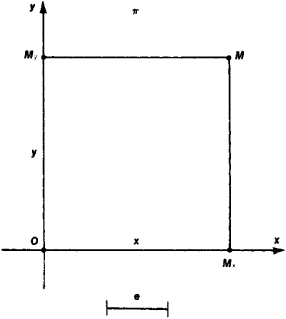Figure 1

unit e, constitute the so-called rectangular Cartesian system of coordinates Oxy in the plane. Straight lines Ox and Oy are called, respectively, the axis of abscissas and the axis of ordinates. The position of any point M in the plane with respect to the system Oxy can be determined in the following manner. Let Mx and My be the projections of M on Ox and Oy and the numbers x and y be the magnitudes of segments OMx and OMv. The magnitude x of segment OMx, for example, is equal to the length of that segment taken with positive sign if the direction from O to Mx coincides with the direction on straight line Ox and with negative sign in the opposite case. The numbers x and y are said to be the rectangular Cartesian coordinates of point M in the system Oxy. Usually they are referred to as the abscissa and the ordinate of point M, respectively. The symbol M (x, y) is used to designate a point M having abscissa x and ordinate y. It is evident that the coordinates of point M define its position with respect to system Oxy.

Let L be a certain curve on plane π with a given rectangular Cartesian coordinate system Oxy. Using the idea of the point coordinates, it is possible to introduce the concept of an equation of the given curve L with respect to system Oxy in the form of a relationship of type F (x, y) = 0 which is satisfied by the coordinates x and y of any point M located on L and not satisfied by any point which does not lie on L. If, for example, curve L is a circle of radius R with the center at the origin of coordinates O, the equation x2 + y2R2 = 0 will be the equation of the circle, as is evident from Figure 2. If point M lies on the circle, then from the Pythagorean will be the equation of the circle, as is evident from Figure 2. If point M lies on the circle, then from the PythagoreanFigure 2

theorem we have x2 + y2R2 = 0 for triangle OMMx. If, however, the point does not lie on the circle, then it is clear that x2 + y2R2 ≠ 0. Thus, curve L in the plane can be associated with its equation F (x, y) = 0 with respect to coordinate system Oxy.

The basic idea behind the method of plane coordinates is that the geometric properties of curve L can be investigated through the study by analytical and algebraic means of the properties of F (x, y) = 0, the equation of the curve. For example, let us apply the method of coordinates to ascertain the number of points of intersection between circle C with radius R and a given straight line B (Figure 3). Let the origin of coordinates of Oxy lie at the center of the circle, and let axis Ox be perpendicular to straight line B. Since straight line B is perpendicular to axis Ox, the abscissa of any point on that line is equal to a certain constant a. Thus, the equation of straight line B will have the form xa = 0. The coordinates (x, y) of the point of intersection of circle C (whose equation has the form x2 + y2R2 = 0) and straight line B satisfy both equations

(1) x2 + y2R2 = 0, a = 0

that is, they constitute a solution of equations (1). Consequently, the geometrical question of the number of points of intersection of a straight line and a circle is reduced to the analytical question of the number of solutions for the algebraic system of equations (1). Solving that system, we arrive at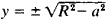. Thus, the circle and the straight line may intersect at two points (R2 > a2) as shown in Figure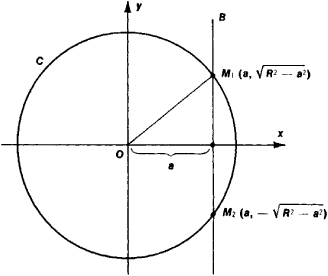Figure 3

3; may have one common point (R2 = a2), in which case the straight line B is tangent to circle C; or may have no common points (R2 < a2), in which case straight line B lies outside circle C.

Analytic geometry studies in detail the geometrical properties of the ellipse, the hyperbola, and the parabola, which are the curves of intersection of a circular cone with planes that do not pass through the apex of the cone. These curves are frequently encountered in many problems in natural science and technology. For example, the motion of a material point under the influence of a central gravity field follows one of these curves; engineering in the construction of projectors, antennas, and telescopes uses the important optical property of the parabola in which light rays proceeding from a certain point, called the focus of the parabola, following reflection from the parabola form a parallel beam.

In analytic geometry, a systematic study is made in the plane of the so-called algebraic curves of first- and second-order; these lines in rectangular Cartesian coordinates are defined with first- and second-degree algebraic equations, respectively. First-order curves are straight, and conversely, every straight line is defined by the first-degree equation Ax + By + C = 0. Second-order curves are defined by equations of type, Ax + Bxy + Cy2 + Dx + Ey + F = 0. The basic method of analyzing and classifying these curves consists in selecting a rectangular Cartesian coordinate system in which the equation of the curve will have the simplest possible form and in the subsequent study of this simple equation. It can be demonstrated that by such means the equation of any real second-order curve can be reduced to one of the following simplest forms:The first of these equations defines an ellipse, the second a hyperbola, the third a parabola, and the last two a pair of straight lines (intersecting, parallel, or coincident).

In analytic geometry the method of coordinates is also used in space. Here, the rectangular Cartesian coordinates x, y, and z (abscissa, ordinate, and Z-coordinate) of point M are completely analogous to the plane case (Figure 4). Each surface S in space can be associated with an equation F (x, y, z) =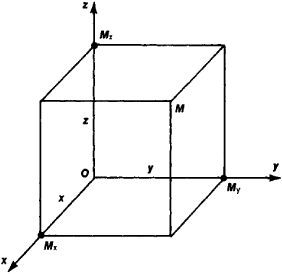Figure 4

0 with respect to coordinate system Oxyz. For example, the equation of a sphere of radius R with center at the origin of coordinates has the form x2 + y2 + z2R2 = 0. Here, the geometrical properties of surface 5 are determined by studying algebraically and analytically the properties of the equation of this surface. The curve L in space is given as the curve of intersection of the two surfaces S1 and S2. If F1(x, y, z) = 0 and F2 (x, y, z) = 0 are the equations of S1and S2, then these two equations taken together constitute the equation of curve L. For example, straight line L in space can be regarded as the line of intersection of two planes. Since a plane in space is defined by an equation of the form Ax + By + Cz + D = 0, a pair of equations of this same type considered together is the equation of straight line L. The method of coordinates, therefore, can be applied to the study of curves in space. Analytic geometry in space systematically studies the so-called algebraic surfaces of the first and second order. Algebraic surfaces of the first order, clearly, can only be planes. Surfaces of the second order are defined by equations of the form

Ax2 + By2 + Cz2 + Dxy + Eyz + Fxz + Gx + Hy + Mz + N = 0

The basic method of studying and classifying these surfaces consists in the selection of a rectangular Cartesian coordinate system in which the equation of the surface has the simplest possible form and in the subsequent study of this simple equation. The most important real second-order surfaces are the ellipsoid, the hyperboloids of one and two sheets, and the elliptic and hyperbolic paraboloids. These surfaces in specially chosen rectangular Cartesian coordinate systems have the following equations: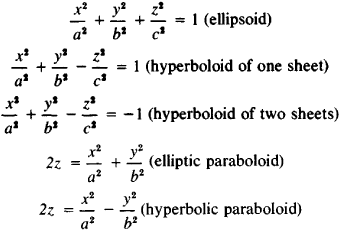The most important surfaces of the second order are encountered frequently in various problems in mechanics, solid-state physics, theoretical physics, and engineering. Thus, in the study of stresses arising in solid bodies, use is made of the concept of the so-called stress ellipsoid. Designs in the form of hyperboloids and paraboloids are used in various engineering projects.

### REFERENCES

Descartes, R. Geometriia. Moscow-Leningrad, 1938. (Translated from French.)
Wileitner, H. Istoriia matematiki ot Dekarta do serediny XIX stoletiia, 2nd ed. Moscow, 1966. (Translated from German.)
Efimov, N. V. Kratkii kurs analiticheskoi geometrii, 9th ed. Moscow, 1967.
Il’in, V. A., and E. G. Pozniak. Analiticheskaia geometriia. Moscow, 1967.
Aleksandrov, P. S. Lektsii po analiticheskoi geometrii. Moscow, 1968.
Bakhvalov, S. V., P. S. Modenov, and A. S. Parkhomenko. Sbornik zadach po analiticheskoi geometrii, 3rd ed. Moscow, 1964.
Kletenik, D. V. Sbornik zadach po analiticheskoi geometrii, 9th ed. Moscow, 1967.

E. G. POZNIAK

## analytic geometry

[‚an·əl′id·ik jē′äm·ə·trē]
(mathematics)
The study of geometric figures and curves using a coordinate system and the methods of algebra. Also known as cartesian geometry.
McGraw-Hill Dictionary of Scientific & Technical Terms, 6E, Copyright © 2003 by The McGraw-Hill Companies, Inc.
References in periodicals archive ?
We use the rules of analytic geometry. We locate the square in a system of coordinates in such a manner that the vertex A coincides with the origin and the sides AB and AD lie on the axes x and y, respectively (see Figure 13).
Based on the principle of space analytic geometry, the equations of the cylinder and the pipes are given as
Moreover, to articulate the structure of analytic geometry the following six aspects of mathematical thinking are very crucial: (1) Generalization (2) Analysis and analytical thinking (3) Logical thinking (4) Abstract thinking (5) Problem solving (6) Representation.As, algebraic concepts are everywhere in school geometry and, it is a best approach to the solution of problem in geometry by involving and understanding the concepts of variables (Dindyal, 2007).
I was pleasantly surprised that the book also provides a summary of a number of the concepts that students may need prior to tackling the problems; for example, the section on Analytic Geometry provides a quick summary on the equations of lines, parabolas and circles, distance and slope between two points.
(7) It is an easy exercise in high school analytic geometry to define, given an R-scale on a manifold, a one-dimensional (one-coordinate) scale on any line of the manifold, and conversely to extend a one-dimensional scale on a line to a three-dimensional scale on the whole manifold.
These courses (covering Grades 8 to 12) included low Grade 8 mathematics, average Grade 8 mathematics, basic mathematics, vocational mathematics, consumer mathematics, NEC mathematics, geometry (including honors), prealgebra, algebra I (including honors), algebra II (including honors), trigonometry (including honors), analytic geometry (pre-calculus), and calculus.
Descartes ought to be discussed, given his connection with analytic geometry; yet, this book is supposed to be about Khayyam.
The comprehensive software includes Euclidian, transformational and analytic geometry, and students and teachers report it's easy to learn and use.
The BEC in the magnetic trap is ellipsoidal in shape and the required modifications are straightforward applications of analytic geometry.
College-prep students and teachers can work on problems in algebra, trigonometry, analytic geometry and calculus.
This paper reports the results of student use of a TI-92 graphing calculator to solve algebra and analytic geometry problems in 9th grade Austrian classes.

Site: Follow: Share:
Open / Close